• 对偶问题基本性质
千次阅读
2021-07-09 22:28:50更多相关内容
• 每个线性规划问题都有一个与之对应的对偶问题对偶问题是以原问题的约束条件和目标函数为基础构造而来的，在求出一个问题解的时候，也同时给出了另一问题的解。在某些情况下，利用对偶理论求解线性规划问题会更为...

经过前两期的学习，想必大家已经对线性规划问题有了详细的了解。本期，小编将带大家学习线性规划问题的对偶问题理论。

每个线性规划问题都有一个与之对应的对偶问题，对偶问题是以原问题的约束条件和目标函数为基础构造而来的，在求出一个问题解的时候，也同时给出了另一问题的解。在某些情况下，利用对偶理论求解线性规划问题会更为简单，而且有助于深入了解待求问题的本质。

通过对线性规划对偶理论基础知识的梳理和总结，小编绘制了《线性规划对偶理论与灵敏度分析思维导图》，如下所示。线性规划对偶理论与灵敏度分析章节一共有6个知识点和15个子知识点。6个知识点分别是线性规划的对偶问题、对偶问题的基本性质、影子价格、对偶单纯形法、灵敏度分析以及参数线性规划。

·第1个知识点是线性规划的对偶问题，该部分有3个子知识点，包括线性规划的对偶问题概述、对称形式下对偶问题的一般形式以及非对称形式的原-对偶问题关系；

·第2个知识点是对偶问题的基本性质，我们利用对偶问题的基本性质可以快速得到对偶问题或原问题的解。该部分共包括5个子知识点，首先是单纯形法计算的矩阵描述，为后面的性质介绍做铺垫，之后就是对偶问题的4个基本性质：弱对偶性、最优性、对偶定理以及互补松驰性；

·第4个知识点是对偶单纯形法，对偶单纯形法是指从对偶可行性逐步搜索出原始问题最优解的方法，该节主要有2个子知识点，分别是对偶单纯形法的基本思路和计算步骤；

·第5个知识点是灵敏度分析，之前讲到的线性规划问题中的参数都是已知常数，但实际上往往需要估计和预测，对偶理论的灵敏度分析就是为了解决参数估计的问题，该节包含2个子知识点，分别是灵敏度分析的步骤与参数改变的不同情况分析；

·第6个知识点是参数线性规划，参数线性规划(parametric linear programming)是数学规划的一个分支，它的研究系数不是常数，而是在某范围内变化的参数的线性规划问题。该节包含2个子知识点，分别是参数线性规划的形式与问题分析步骤。

今天，小编先带大家学习一下前两个大知识点，即线性规划对偶理论的对偶问题介绍与其基本性质。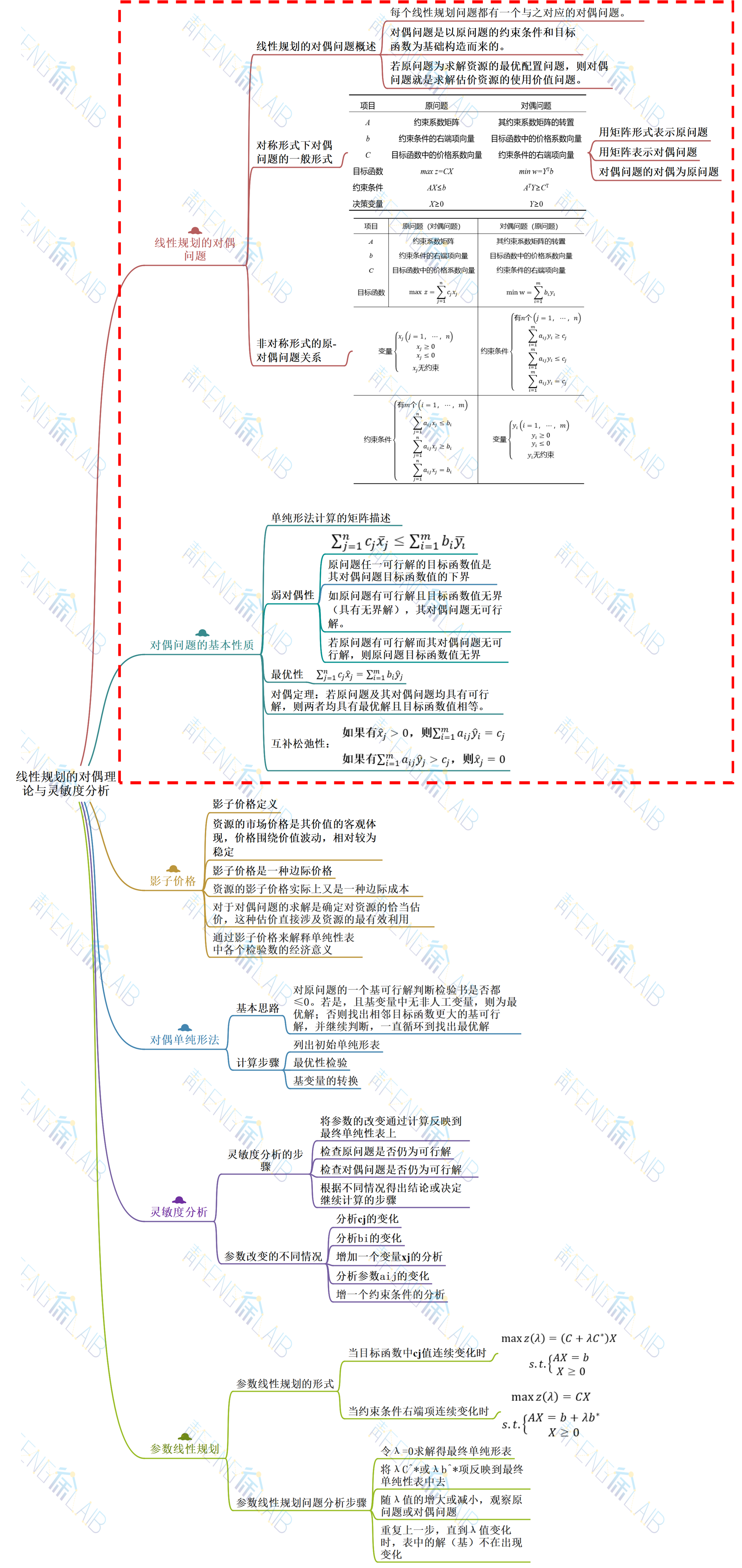一、线性规划的对偶问题

1、对称形式下对偶问题的一般形式对称形式下线性规划原问题的一般形式为：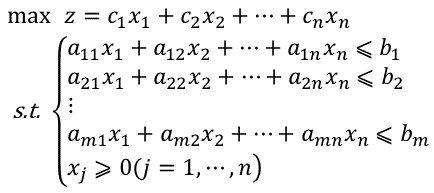其对偶问题的一般形式为：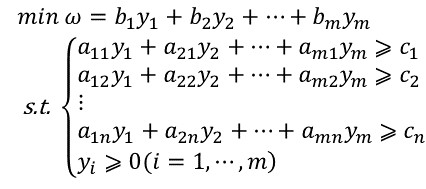用矩阵形和向量形式来表示可写为：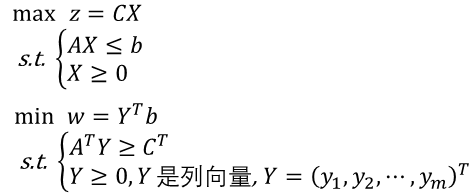我们将上述对称形式下线性规划的原问题与对偶问题进行比较，可以列出如下表所示的对应关系（注：对偶问题的对偶即原问题）：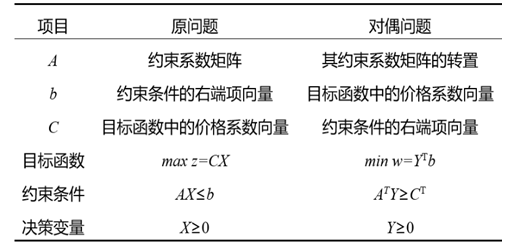2、非对称形式的原-对偶问题关系

因为并非所有线性规划问题具有对称形式，故一般形式下的线性规划问题可以先转换为对称形式，再写出其对偶问题，以下述线性规划问题为例：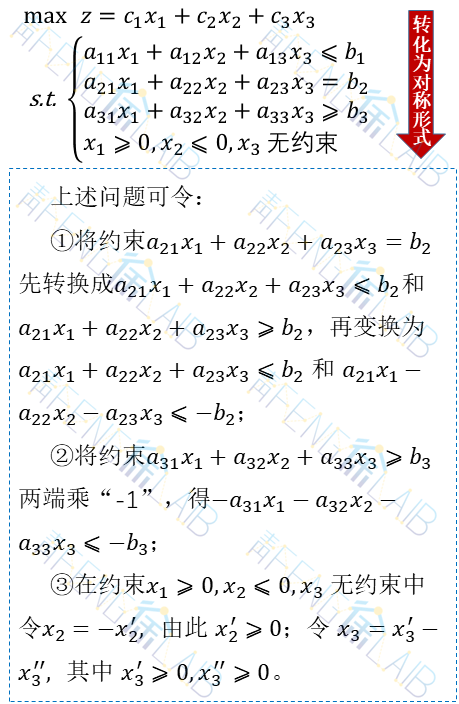经上述变换后线性规划问题可重新表达为：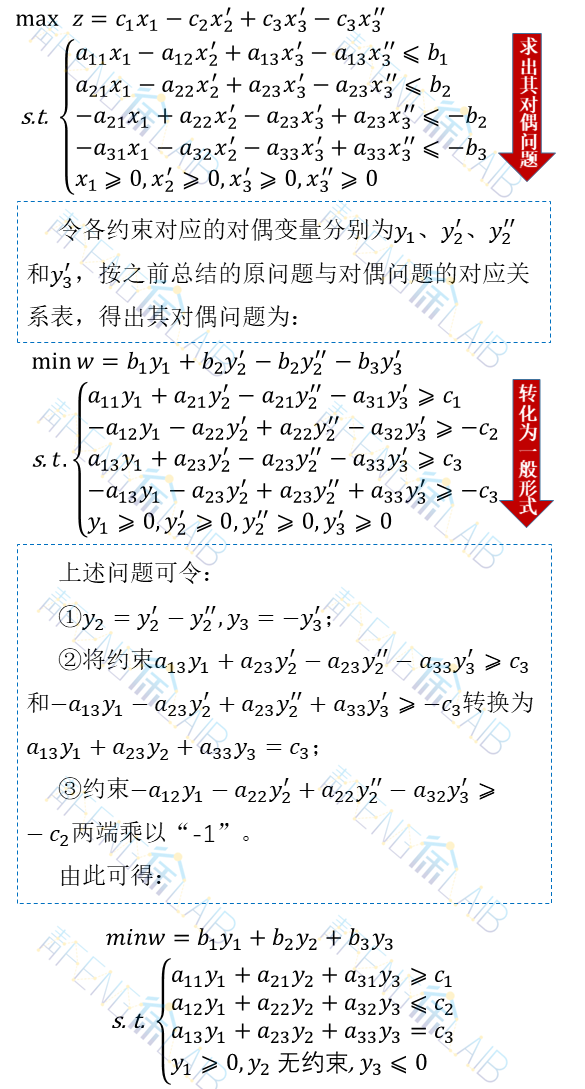我们将对称或不对称线性规划原问题同对偶问题的对应关系，统一归纳为下表所示形式：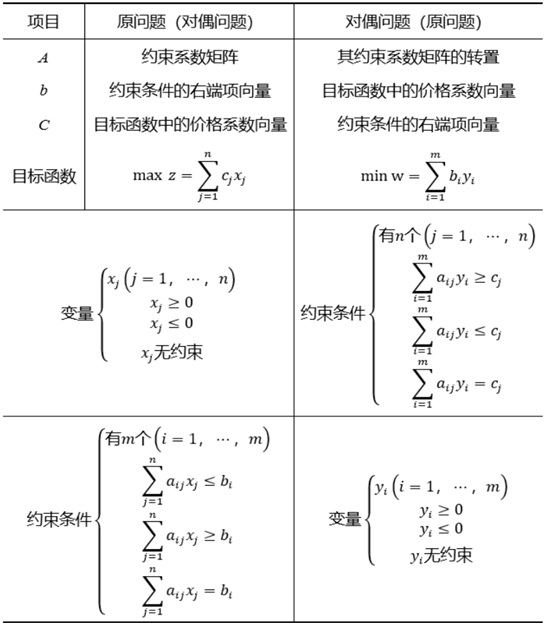二、对偶问题的基本性质

1、单纯形表计算的矩阵描述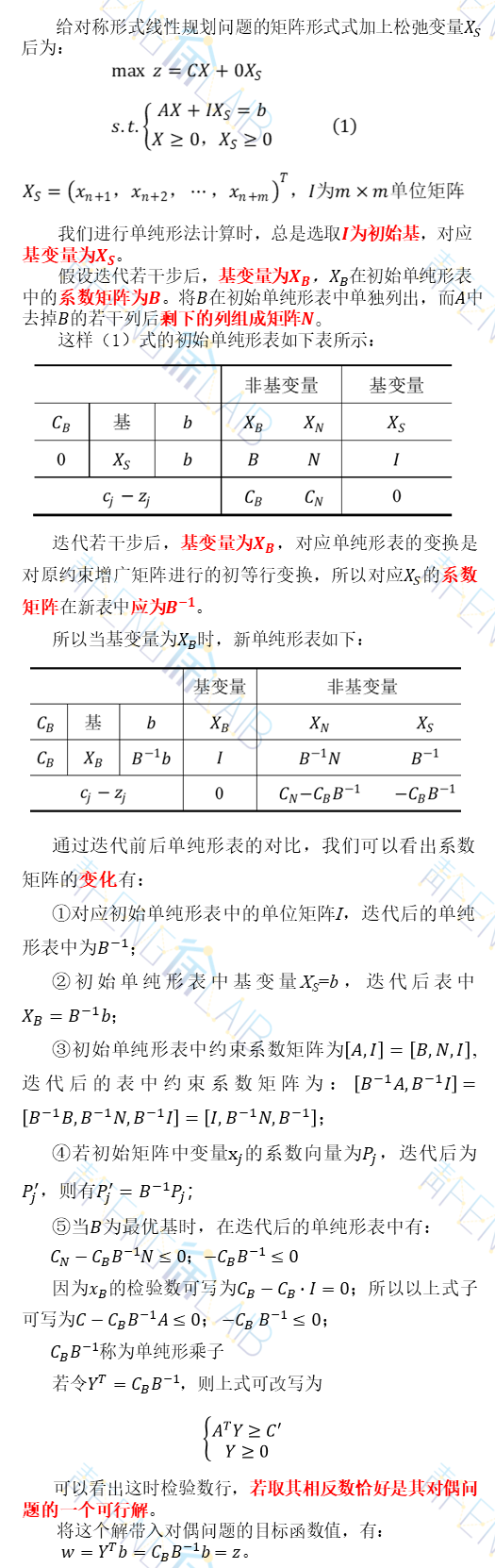由上式看出，当原问题为最优解时，这时对偶问题为可行解，且两者具有相同的目标函数值。根据下一节讲述的对偶问题的基本性质，将看到这时对偶问题的解也为最优解。

这里，小编还专门给大家准备了一个例题，帮助更好的理解原问题及其对偶问题的变量及解之间的对应关系，具体如下所示：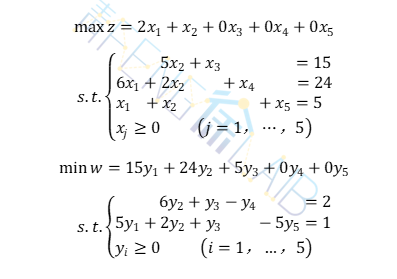两个问题分别的最终单纯形表如下所示：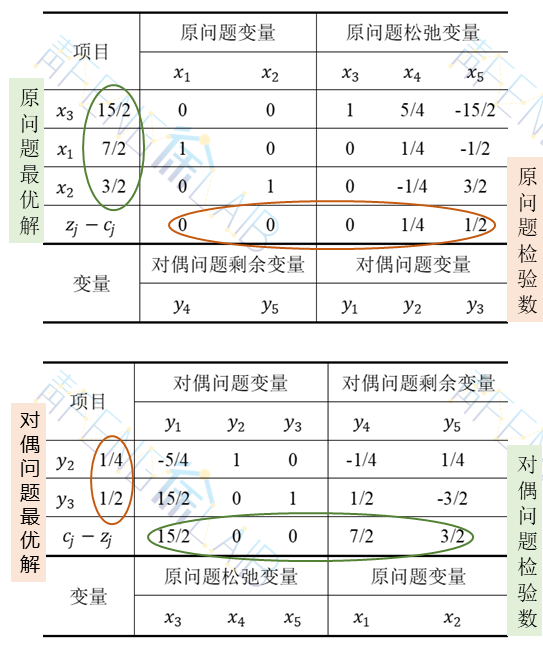原问题与对偶问题的检验数正负不同是由于原问题目标函数是极大值函数，对偶问题目标函数是极小值函数，根据单纯形法计算原理可得原问题检验数为负值，对偶问题检验数为正值。

观察例题中原问题与对偶问题的两张最终单纯形表，我们发现例题中原问题的检验数取相反数即对应对偶问题的最优解，对应了上一节提到的单纯形法矩阵描述中的解的对应关系。

因此，通过例题我们可以直观看出两个问题变量之间的关系，同时看出只需解出其中一个问题，从最优解的单纯形表中可得到另一个问题的最优解。

2、对偶问题的基本性质

在了解了什么是对偶问题之后，小编再带领大家梳理一下对偶问题的基本性质。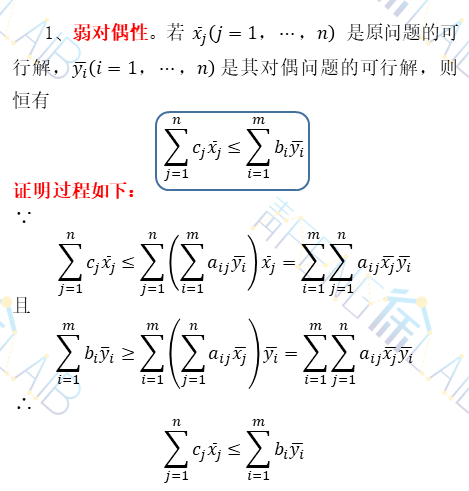弱对偶性是对偶问题非常重要的一个性质，通过弱对偶性，我们可以得出一下推论：

①原问题任一可行解的目标函数值是其对偶问题目标函数值的下界；反之对偶问题任一可行解的目标函数值是其原问题目标函数值的上界。

②如原问题有可行解且目标函数值无界（具有无界解），其对偶问题无可行解；反之对偶问题有可行解且目标函数值无界，则其原问题无可行解（注意哦，本条性质逆不成立，即当对偶问题无可行解时，其原问题或具有无界解或无可行解，反之亦然）。

③若原问题有可行解而其对偶问题无可行解，则原问题目标函数值无界；反之对偶问题有可行解而其原问题无可行解，则对偶问题的目标函数值无界。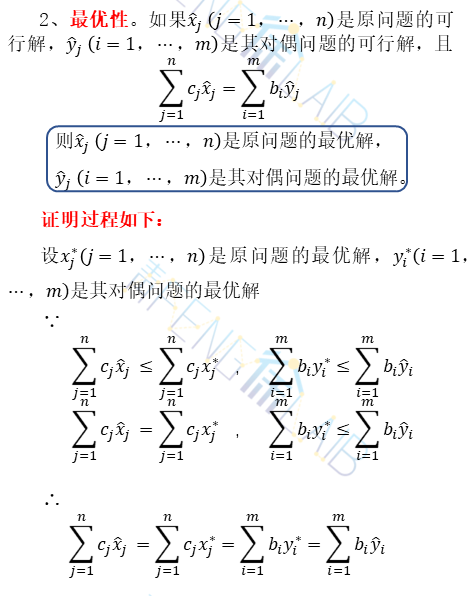（3）强对偶性（或称对偶定理）。若原问题及其对偶问题均具有可行解，则两者均具有最优解且目标函数值相等。

证明过程如下：

∵两者均有可行性，根据弱对偶性的推论①，对原问题的目标函数值具有上界，对偶问题的目标函数值具有下界。

∴两者均具有最优解。

又∵当原问题为最优解时，其对偶问题的解为可行解，且两者目标函数值相同

∴由最优性知，这时两者的解均为最优解。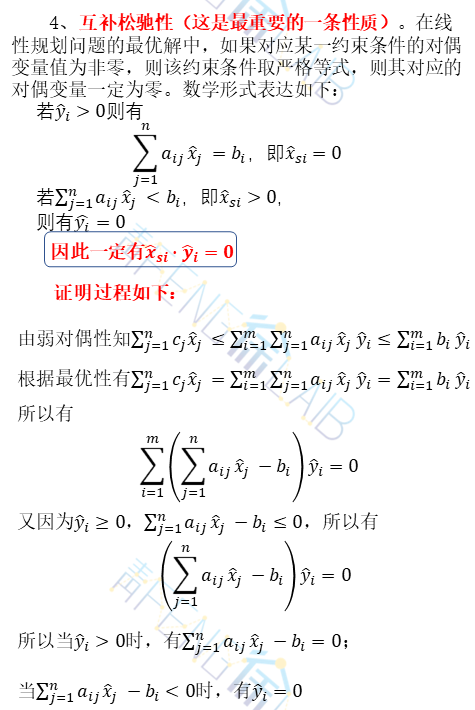对偶问题的四条基本性质小编就介绍完了，这些性质无论是对称形式的对偶问题还是非对称形式的对偶题均适用哦~

展开全文机器学习
• ## 线性规划对偶问题

万次阅读 多人点赞 2021-04-28 23:49:31
对偶问题基本性质五． 对偶问题的单纯形法描述六． 影子价格七． 利用Matlab解决线性规划问题八． 参考SmartyPants创建一个自定义列表如何创建一个注脚注释也是必不可少的KaTeX数学公式新的甘特图功能，丰富你的...

# 线性规划及单纯形法

线性规划是最优化问题的一种特殊情形，实质是从多个变量中选取一组合适的变量作为解，使得这组变量满足一组确定的线性式（约束条件），而且使一个线性函数（目标函数）达到最优

## 〇． 前言

不少人对线性规划问题还只有初高中“图解法”的印象。能使用图像直观明了地解题自然是一种好方法，相信很多人都认为一种好方法够解决需要解决的问题不就足矣了吗。其实我也不例外。这也是我开始接触时的疑惑。但学了一阵子后，我思索了，确实，相比较几何和组合推理，代数学确实在非数学专业的学生看来不够讨喜，尽管代数学相当强大、相当普适……单纯形法就是代数的一个小小缩影，它似乎表面上让线性规划问题变得比“图解法”要复杂得多，但是其超强的普适性着实迷人，我们用计算机来解决线性规划问题可能只要一句编程，但其背后的数学原理却如此丰富。我们光线性规划问题就学了9周（还没上完），可见它背后的数学文化还是相当值得深挖研讨的……<其实为了应试【捂嘴】>
因为单纯形法经过本人预习复习后已经有些开窍了，并成功用大M法解决了我的大作业，所以在此不过多赘述，有兴趣可以去了解【我更倾向于你没兴趣，看完你就知道了】不过鉴于这周要测验，而对偶问题还是迷迷糊糊的我决定开始摸鱼做笔记<说白了就是应试>，希望敲完这篇，我可以把线性规划的对偶理论啃下来吧……<说白了还是为了应试>

果然，第一次还是给了数学……哎嘿~~

## 一． 对偶问题的提出

无论从理论或是实践角度，对偶理论是线性规划中的一个最重要和有趣的概念。支持对偶理论的基本思想是，每一个线性规划问题都存在一个与其对偶的问题，在求出一个问题解的时候，也同时给出了另一问题的解。下面通过实际例子看待对偶问题的经济意义。
<书本上的例子实在是过于晦涩难懂，于是在网上摘了一点好理解的“栗子”……>

【例一】
某工厂用甲乙两种资源生产A、B、C、D四种产品，现有资源数、单位产品所需资源数以及单位产品可获得利润如下表所示。问如何组织生产能够使得利润最大？根据题意列出的线性规划不等式是这样的（大家不用去推出这个公式，目的在于和下文的对偶问题公式形成对比）：但是现在如果从另一个角度考虑问题。假设该厂不生产A、B、C、D四种产品，而是将甲、乙两种资源出租给其他单位，其原则是：识别的单位愿意租，又使本单位获利不低于原利润。问如何给甲、乙两种资源定价最合理？

根据题意列出的线性规划不等式是这样的：可以发现，两个问题下的线性规划公式很相似（具体的如何转换会在下文予以说明）。那么两个问题具有什么样的实际意义呢？可以考虑该厂的目的现在是想要出租资源但是要保证价格不低于资源变成产品所带来的收益。也就是说第二个问题所求出来的最小(优)值应该是第一个问题求出的最大(优)值，换句话说我们可以通过原问题的对偶问题的最优值来获得原问题的最优值，但为什么要这样做呢？直接用原问题来求得最优值不可以吗？这就是我们第二个问题所涉及的了。

【例二】
① ：仔细对比上图两种式子可以发现，图一中的变量较多而且约束条件较少，相信大家都做过线性规划的问题，不难发现变量越少，约束条件越多对于我们的求解就越有利。这里也是这个道理，通过将原问题转换成为其对偶问题，可以使得更加有利于我们求解线性规划问题，并且从问题一的解答中我们了解到两种问题“本是同根生”，所以对偶问题其实是有利于我们计算复杂线性规划问题的一种"辅助"方式。但是，对偶问题一定比原问题变量要少吗？并不是这样的，但是我们可以非常容易的判断出该问题的对偶问题会不会更简单，这个方法涉及到对偶问题的转换，我们在第三个问题中进行解答。
② ：其实有时不仅仅是为了减少变量的个数，有的问题甚至必须要通过转换称为对偶问题才能够解决(博主目前的水平下，非数学专业)，比如为了将原式化成标准式时会出现(不)等式右端出现负数的情况，这时如果仅用单纯形法是不能够解决的，因此从这个角度来看，为应对考试对偶问题是必须要学习的。

【例三】
接下来我们将进入实战，直接用实例来讲解原问题的对偶问题是如何化成的。首先我们以下面这个线性规划问题为例：1. 对偶问题的目标函数和原问题是相反的，原问题是min则对偶问题为max。并且变量的个数也会发生改变，系数是原问题不等式右端的b值(仅仅是化为对偶问题是不需要将原问题化作标准式的)。根据以上得出目标函数：2. 接下来是写约束条件，约束条件的书写是最容易出错的地方。我们先写等式的左端，对偶问题等式的左端是根据原问题等式左端竖着来写的；等式的右端就是直接用原问题目标函数中的系数(先不考虑符号),也就是看如下画红框的部分：3. 根据原问题竖着的系数来作为对偶问题每个等式中变量的系数；原问题目标函数的系数，可以得出如下（先仔细看下红框里的数据是如何得到的）：4. 接着是最为重点的约束条件中的符号和变量的范围符号，这两点是根据如下来进行变换：解释： 根据max类型写min类型的变量符号时，要根据max的约束条件符号，并且与之相反；写min的约束条件符号时，要根据max类型的变量符号，并且与之相同。反之亦然。另外无约束对应的是‘ = ’。最终得到：至此，我们已经讲完了对偶问题的转换方法，下面再举一个max类型转换成min类型的例子，大家可以对照练习加深印象。<因为壳子比较菜又比较摸鱼还不会举“栗子”，所以第一部分的“栗子”都是从优秀博主家摘的……后面有走心原创哦~~>

## 二． 对称形式下对偶问题的一般形式

<你们最爱的 纯数学理论来辣~~~>
定义：满足下列条件的线性规划问题称为具有对称形式：其变量均具有非负约束，其约束条件当目标函数求极大时均取“≤”号，当目标函数求极小时均取“≥”号。
对称形式下线性规划原问题的一般形式为：用yi(i=1,…,m)代替第i种资源的估价，则其对偶问题的一般形式为：用矩阵形式表示，对称问题的线性规划问题的原问题为：其对偶问题为：上述对偶问题中令w’=-w，可改写为：将上述对称形式下线性规划的原问题与对偶问题进行比较，可以列出如下表的对应关系：如将其作为原问题，并按上表所列对应关系写出它的对偶问题则有：再令z=-z’，则上式可改写为：可见对偶问题的对偶即原问题。因此将表中右端的线性规划问题作为原问题，写出其左端形式的对偶问题。

## 三． 非对称形式的原-对偶问题关系

因为并非所有线性规划问题具有对称形式，故下面讨论一般情况下线性规划问题如何写出其对偶问题。考虑下面例子：
<随便举个栗子啊>
【例四】 写出下述线性规划问题的对偶问题：解：

为了写出对偶问题，思路是先将其转化成对称形式，再按上表的对应关系来写。因例中目标函数为max，故约束条件应变换为“≤”号，所有的变量均应为≥0.为此：
<emmm…好像忘了给方程标号了…随手就标，养成好习惯><好了，go on…>

（1）约束条件b两端乘上“-1”；
（2）将约束条件c先等价转换为x1+x2+x3≤4和x1+x2+x3≥4，再变换为x1+x2+x3≤4和-x1-x2-x3≤-4；
（3）令x2’=-x2，所以x2’≥0；
（4）令x3=x3’-x3’’，其中x3’≥0，x3’'≥0。

由此【例四】可变换成具有如下对称形式的线性规划问题：令对应上述4个约束条件的对偶变量分别为y_1,y_2’,y_3’,y_3^’’，按表的对应关系写出其对偶问题为：再令y2=y2’,y3=y3’-y3’’,将第三四个约束条件改为一个等式-5y1-6y2’+y3=3，于是有：将上述对偶问题同【一】的原问题对比发现，无论对称或非对称的线性规划问题在写出其对偶向题时，表中前4行的对应关系都适用，区别的只是约束条件的形式与其对应变量的取值。根据本例中约束和变量的对应关系，下面将对称或不对称线性规划原问题同对偶问题的对应关系，统一归纳为下表所示形式：<看到这里是不是感jio要吐了……而这才只到如何写线性规划的对偶问题，你还没解呢……急啥，快到性质了……>

## 四． 对偶问题的基本性质

<这里才是你真正应该感jio到要吐的地方…>
<还是决定手写，这里敲公式多半会哭死……>

1. 弱对偶性·弱对偶性的推论：
（1） 原问题任一可行解的目标函数值时其对偶问题目标函数值的下界；反之对偶问题任一可行解的目标函数值是其原问题目标函数值的上界。<有点儿绕，但不是不好理解>
（2） 如原问题有可行解且目标函数值无界（具有无界解），则其对偶问题无可行解；反之对偶问题有可行解且目标函数值无界，则原问题无可行解（注意：本点性质的逆不成立，当对偶问题无可行解时，其原问题或具有无界解或无可行解，反之亦然）。
（3） 若原问题有可行解而其对偶问题无可行解，则原问题目标函数值无界；反之对偶问题有可行解而其原问题无可行解时，其对偶问题的目标函数值无界。

2. 最优性3. 强对偶性（或称对偶原理）

若原问题及其对偶问题均具有可行解，则两者均具有最优解，且它们最优解的目标函数值相等。

由于两者均有可行解，根据弱对偶性的推论（1），对原问题的目标函数值具有上界，对偶问题的目标函数值具有下界，因此两者均具有最优解。当原问题为最优解时，其对偶问题的解为可行解，且有z=w，由最优性知，这时两者的解均为最优解。

4. 互补松弛性5.基解互补性
原问题及其对偶问题之间存在一对互补的基解，其中原问题的松弛变量对应对偶问题的变量对偶问题的剩余变量对应原问题的变量.这些互相对应的变量如果在一个问题的解中是基变量则在另一个问题的解中是非基变量；将这对互补的集解分别带入原问题和对偶问题的目标函数中有 z = w

## 五． 对偶问题的单纯形法描述

<听嗦考试必考……但其实我并不熟悉，慌~~>
既然是只菜狗，那就参考一下大佬的吧：

参考链接：
对偶问题的单纯形法

## 六． 影子价格

首先，我们先要认识到互补松弛性的作用：
① 简化求对偶问题最优解过程 : 已知一个线性规划问题的最优解 , 可以 简化求另外一个问题最优解的过程 , 避免使用两次单纯形法求解 ;
② 影子价格问题 : 使用互补松弛定理可以进行一些 经济解释 , 如影子价格问题 ;
影子价格 是 对偶问题的 经济解释 ;影子价格是对偶问题的变量值
<懒了，不想举“栗子”了，有关边际价格的“栗子”还挺多的，有兴趣可以在*“参考”*里摘摘>

## 七． 利用Matlab解决线性规划问题

因为毕竟是计算机系的在读小学生，那就提一嘴实战应用咯。因为linprog这个函数比较常用，也是建模赛事中最最最基础的数学模型了，所以对于大家也不陌生。不过你想要用好它可不止单纯会敲一个linprog那么easy，至少你要知道线性规划的标准型，这样才能套对参数，合理求解线性规划问题。
所以简单截个图：<就是懒？不（shi）是（de）>### 八． 参考

链接: https://blog.csdn.net/qq_43539633/article/details/109150749
链接: https://blog.csdn.net/PursueLuo/article/details/112251520
链接: https://blog.csdn.net/weixin_43848054/article/details/105748797
链接: https://blog.csdn.net/shulianghan/article/details/112096559
《运筹学教程》 胡运权 郭耀煌

展开全文• 给定一个二分类问题的训练样本集D={(x1,y1),(x2,y2),...,(xm,ym)},yi∈{−1,+1}D={(x1,y1),(x2,y2),...,(xm,ym)},yi∈{−1,+1}D=\{(\boldsymbol{x}_1,y_1),(\boldsymbol{x}_2,y_2),...,(\boldsymbol{x}_m,y_m)\},y_i...

#原问题提出
给定一个二分类问题的训练样本集 D = { ( x 1 , y 1 ) , ( x 2 , y 2 ) , . . . , ( x m , y m ) } , y i ∈ { − 1 , + 1 } D=\{(\boldsymbol{x}_1,y_1),(\boldsymbol{x}_2,y_2),...,(\boldsymbol{x}_m,y_m)\},y_i\in \{-1, +1\} ，我们的目标是找到一条分界线/超平面来将两类区分开，如下图：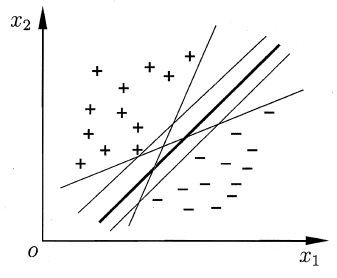在样本空间中，超平面可以通过以下线性方程来描述：
w T x + b = 0 \boldsymbol{w}^T\boldsymbol{x}+b=0
其中， w = ( w 1 , w 2 , . . . , w d ) \boldsymbol{w}=(w_1,w_2,...,w_d) 为法向量，决定了超平面的方向； b b 为位移项，决定了超平面与原点的距离。

如果一个超平面能够将训练样本正确分类，则我们希望这个超平面具有的性质是，对于 ( x i , y i ) ∈ D (\boldsymbol{x}_i,y_i)\in D ，有
\boldsymbol{w}^T\boldsymbol{x}+b\left{ \begin{aligned} 0,\ y_i=+1 \ <0,\ y_i=-1 \end{aligned} \right.
这个式子也是我们在得到超平面之后预测一个新样本的判决条件。

显然，上图中 5 个超平面都满足要求。但是，哪一个是最好的呢？

直观上看，应该去找位于两类训练样本“正中间”的超平面，即上图中最粗的分界线。因为这条线能够在最大程度上容忍两类的数据波动和噪声。

因此，为了能够得到“正中间”位置的超平面，我们将上述条件变得更加严格：
w T x + b { ≥ + 1 ,   y i = + 1 ≤ − 1 ,   y i = − 1 ( 1 ) \boldsymbol{w}^T\boldsymbol{x}+b\left\{ \begin{aligned} \ge+1,\ y_i=+1 \\ \le-1,\ y_i=-1 \end{aligned} \right. \qquad(1)
显然，条件严格后，靠近样本边缘的一些超平面可能会不符合要求，最后会剩下比较靠中间的几个超平面。
那么，如何选出最“正中间”的那个超平面呢？我们可以给一个具体的量化目标：让超平面与最近的样本的距离最大。

如下图所示，距离超平面最近的这几个样本点可以使得公式 (1) 的等号成立，它们被称为“支持向量”（Support Vector）。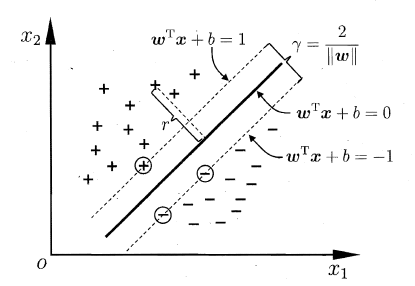样本空间中任意点 x \boldsymbol{x} 到超平面的距离的计算公式为：
r = ∣ w T x + b ∣ ∣ ∣ w ∣ ∣ r=\frac{|\boldsymbol{w}^T\boldsymbol{x}+b|}{||\boldsymbol{w}||}
因此，两类的支持向量到超平面的距离之和为
γ = 2 w , \gamma =\frac{2}{\boldsymbol{w}},
它被称为“间隔”。

如果想找到具有“最大间隔”（maximum margin）的超平面，那么需要最大化 γ \gamma ，也就是需要最小化 ∣ ∣ w ∣ ∣ 2 ||\boldsymbol{w}||^2 。因此，最后需要求解的问题为：
max ⁡ w , b   1 2 ∣ ∣ w ∣ ∣ 2 s . t . y i ( w T x + b ) ≥ 1 , i = 1 , 2 , . . . , m ( 2 ) \begin{aligned} \max_{\boldsymbol{w},b}\ &amp;\quad \frac{1}{2}||\boldsymbol{w}||^2 \\ s.t. &amp;\quad y_i(\boldsymbol{w}^T\boldsymbol{x}+b)\ge1,\quad i=1,2,...,m \end{aligned} \qquad (2)
这就是支持向量机（Support Vector Machine,SVM）的基本型。

# 对偶问题推导

我们希望求解问题 (2) 来得到“正中间”的分界超平面：
f ( x ) = w T x + b f(\boldsymbol x)={\boldsymbol w}^T\boldsymbol x + b
其中， w \boldsymbol w b b 是模型参数，正是问题 (2) 的最优解。问题 (2) 本身是一个凸二次规划问题，可以直接使用现成的优化计算包求解。但我们有更高效的办法，即转为求解其对偶问题，转换过程如下。

根据拉格朗日乘子法，对原问题的每条约束添加拉格朗日乘子 α ≥ 0 \alpha \ge0 ，则该问题的拉格朗日函数可写为：
L ( w , b , α ) = 1 2 ∣ ∣ w ∣ ∣ T + ∑ i = 1 m α i [ 1 − y i ( w T x i + b ) ] ( 3 ) L(\boldsymbol w,b,\boldsymbol\alpha)=\frac1 2 ||\boldsymbol w||^T+\sum_{i=1}^{m}\alpha_i[1-y_i(\boldsymbol w^T\boldsymbol x_i+b)]\quad(3)
其中 α = ( α 1 , α 2 , . . . , α m ) 。 \boldsymbol\alpha=(\alpha_1,\alpha_2,...,\alpha_m)。
可发现
α i [ 1 − y i ( w T x i + b ) ] ≤ 0 \alpha_i[1-y_i(\boldsymbol w^T\boldsymbol x_i+b)]\le0
所以 L ( w , b , α ) ≤ 1 2 ∣ ∣ w ∣ ∣ T L(\boldsymbol w,b,\boldsymbol\alpha)\le \frac1 2 ||\boldsymbol w||^T ，即拉格朗日函数是原问题的一个下界。我们要想找到最接近原问题最优值的一个下界，就需要求出下界的最大值。

根据拉格朗日对偶性，原始问题的对偶问题是最大化最小问题：
max ⁡ α min ⁡ w , b L ( w , b , α ) \max_\alpha \min_{\boldsymbol{w},b}L(\boldsymbol w,b,\boldsymbol\alpha)

首先，求 min ⁡ w , b L ( w , b , α ) \min_{\boldsymbol{w},b}L(\boldsymbol w,b,\boldsymbol\alpha) ，消除掉 w , b \boldsymbol w,b ：分别令 w , b \boldsymbol w,b 的偏导为0，可推出
∂ L ∂ w = w + ∑ i = 1 m α i ( − y i x i ) = 0   ⇒ w = ∑ i = 1 m α i y i x i ( 4 ) ∂ L ∂ w = − ∑ i = 1 m α i y i = 0     ⇒ ∑ i = 1 m α i y i = 0   ( 5 ) \begin{aligned} \frac{\partial L}{\partial \boldsymbol w}&amp;=\boldsymbol w + \sum_{i=1}^m \alpha_i(-y_i \boldsymbol x_i)=0 \ \Rightarrow \boldsymbol w=\sum_{i=1}^m \alpha_i y_i \boldsymbol x_i\quad(4)\\ \frac{\partial L}{\partial \boldsymbol w}&amp;= -\sum_{i=1}^m \alpha_i y_i=0 \qquad\quad\ \ \ \Rightarrow \sum_{i=1}^m \alpha_i y_i=0\ \quad(5) \end{aligned}
将公式（4）（5）带入到拉格朗日函数(3)，得到
L ( w , b , α ) = 1 2 ∣ ∣ w ∣ ∣ T + ∑ i = 1 m α i [ 1 − y i ( w T x i + b ) ] = 1 2 w T w + ∑ i = 1 m α i − ∑ i = 1 m α i y i x i T w − ∑ i = 1 m α i y i b = 1 2 w T w + ∑ i = 1 m α i − w T w − 0 = ∑ i = 1 m α i − 1 2 ∑ i = 1 m ∑ j = 1 m α i α j y i y j x i T x j T \begin{aligned} L(\boldsymbol w,b,\boldsymbol\alpha) &amp;=\frac1 2 ||\boldsymbol w||^T+\sum_{i=1}^{m}\alpha_i[1-y_i(\boldsymbol w^T\boldsymbol x_i+b)]\\ &amp;=\frac1 2 \boldsymbol w^T\boldsymbol w +\sum_{i=1}^m\alpha_i -\sum_{i=1}^m\alpha_iy_i\boldsymbol x_i^T\boldsymbol w-\sum_{i=1}^m\alpha_iy_ib\\ &amp;= \frac 1 2\boldsymbol w^T \boldsymbol w+\sum_{i=1}^m\alpha_i-\boldsymbol w^T\boldsymbol w-0\\ &amp;=\sum_{i=1}^m\alpha_i-\frac1 2 \sum_{i=1}^m\sum_{j=1}^m \alpha_i\alpha_jy_iy_j\boldsymbol x_i^T\boldsymbol x_j^T \end{aligned}
因此，问题（2）的对偶问题是
max ⁡ α ∑ i = 1 m α i − 1 2 ∑ i = 1 m ∑ j = 1 m α i α j y i y j x i T x j T ( 6 ) s . t . ∑ i = 1 T α i y i = 0 α i ≥ 0 , i = 1 , 2 , . . . , m . \begin{aligned} \max_\alpha\quad &amp;\sum_{i=1}^m\alpha_i-\frac1 2 \sum_{i=1}^m\sum_{j=1}^m \alpha_i\alpha_jy_iy_j\boldsymbol x_i^T\boldsymbol x_j^T\quad(6)\\ s.t.\quad&amp;\sum_{i=1}^T\alpha_iy_i=0\\ &amp;\alpha_i\ge0,i=1,2,...,m. \end{aligned}
解出 α \boldsymbol \alpha 后，求出 w \boldsymbol w b b

（1） w \boldsymbol w 可以由公式（4）得到。

（2） b b 可以根据一个支持向量必定会满足的条件得到： y i ( w T x i + b ) = 1 y_i({\boldsymbol w}^T\boldsymbol x_i + b)=1 ，因此如果第 k k 个样本是一个支持向量（即 α k &gt; 0 \alpha_k&gt;0 ），可以得到 b = y k − w T x k b=y_k-{\boldsymbol w}^T\boldsymbol x_k 。不过，经常会将所有的支持向量都计算出对应的 b b 来，然后取均值：
b = 1 n s ∑ i = 1 m ( y i − w T x i ) I ( α i &gt; 0 ) b=\frac{1}{n_s}\sum_{i=1}^m(y_i-{\boldsymbol w}^T\boldsymbol x_i)I(\alpha_i&gt;0)
其中， n s n_s 为支持向量的个数，即 n s = c o u n t ( α i &gt; 0 ) n_s=count(\alpha_i&gt;0)
综上，可以得到分界线：
f ( x ) = w T x + b = ∑ i = 1 m α i y i x i T x + 1 n s ∑ i = 1 m ( y i − w T x i ) I ( α i &gt; 0 ) \begin{aligned} f(\boldsymbol x)&amp;=\boldsymbol w^T\boldsymbol x + b\\ &amp;=\sum_{i=1}^m\alpha_iy_i\boldsymbol x_i^T\boldsymbol x+\frac{1}{n_s}\sum_{i=1}^m(y_i-{\boldsymbol w}^T\boldsymbol x_i)I(\alpha_i&gt;0) \end{aligned}
需要注意的是，问题（2）有不等式约束，因此对偶问题需要满足KKT条件：
{ α i ≥ 0 y i f ( x i ) − 1 ≥ 0 α i ( y i f ( x i ) − 1 ) = 0 \left\{ \begin{aligned} &amp;\alpha_i\ge0 \\ &amp;y_if(\boldsymbol x_i)-1\ge0\\ &amp;\alpha_i(y_if(\boldsymbol x_i)-1)=0 \end{aligned} \right.
因此，对于训练样本 ( x i , y i ) (\boldsymbol x_i,y_i) ，总有 α i = 0 \alpha_i=0 y i f ( x i ) = 1 y_if(\boldsymbol x_i)=1 。若 α i = 0 \alpha_i=0 ，则该样本不会对 f ( x ) f(\boldsymbol x) 的值有任何影响；若 α i &gt; 0 \alpha_i&gt;0 ，则必有 y i f ( x i ) = 1 y_if(\boldsymbol x_i)=1 ，所对应的样本点位于最大间隔边界上，是一个支持向量。这显示出支持向量的一个重要性质：训练完成后，大部分的训练样本都不需要保留，最终模型仅与支持向量有关。

展开全文SVM
• C T Y ≥ 0 \begin{array}{lcl} \rm minW = b^T Y \\\\ \rm s.t\begin{cases} \rm A^TY \geq C^T \\\\ \rm Y \geq 0 \end{cases}\end{array} minW=bTYs.t⎩⎪⎨⎪⎧​ATY≥CTY≥0​​ 由对偶问题基本性质得到如下...
• 对偶理论总结 ( 对称性质 | 弱对偶定理 | 最优性定理 | 强对偶性 | 互补松弛定理 )
• SVM目前被认为是最好的现成的分类器，SVM整个原理的推导过程也很是复杂啊，其中涉及到很多概念，如：凸优化问题、拉格朗日乘子法、对偶问题，slater条件、KKT条件还有复杂的SMO算法！相信有很多研究过SVM的小伙伴们...
• 线性规划的对偶问题与原问题互为对偶，线性规划的原问题对偶问题地位具有对称关系。 原问题对偶问题的转化 例子 原问题对偶问题： 转化方法 1、原问题有几个约束，对偶问题就有几个变量 2、原来的约束...
• ## 强对偶性与KKT条件

千次阅读 2021-08-11 21:40:31
对偶性意味着原问题对偶问题的最优值达到相等，没有对偶间隙。 强对偶性不总是成立（即使是对于凸问题）。凸问题usually (but not always)有强对偶性。 有很多条件使强对偶性成立，这些条件称为constraint ...凸函数 kkt条件
• 对偶问题基本性质 (1) 对称性 对偶问题对偶是原问题。 (2) 弱对偶性 m i n ( - ω′) = - C X ; - A X ≥ - b ; X ≥ 0 m i n ( - ω′) = m a x ω′ m a x ω′= m a x z = C X ; A X ≤ b ; X ≥...
• 线性规划中一个经典问题的描述如下： 　某工厂有两种原料A、B，而且能用其生产两种产品： 1、生产第一种产品需要2个A和4个B，能够获利6； 2、生产第二种产品需要3个A和2个B，能够获利4； 此时共有100个A和...
• 转自西瓜书《机器学习》上节我们介绍了支持向量机的基本型及其推导过程，这节我们对基本型求解。上节我们推导的模型为我们希望求解上式来得到大间隔划分超平面所对应的模型其中w，b是模型参数，注意到式（1）本身是...SVM
• 每个线性规划问题都有一个与之对应的对偶问题对偶问题也是一个线性规划问题，并且对偶问题对偶问题是原问题。原问题的最优解可以由对偶问题得到，有时候利用对偶理论求解线性规划问题更加简单，也更能了解问题的...
• 首先，给出了B-（p，r）-预不变凸函数的几个基本性质，表明B-（p，r）-预不变凸函数仍然满足加法，数乘和复合函数运算性质，并举例说明了B-（p，r）-预不变凸函数是B-（p，r）-不变凸函数的真推广。然后，重点讨论了...
• http://course.cug.edu.cn/cugFirst/operational_research/main/charpter2/p2.htm 转载于:https://www.cnblogs.com/macula7/archive/2009/05/18/1960820.html
• 本文承接上一篇 约束优化方法之拉格朗日乘子法与KKT条件，将详解一些拉格朗日对偶的内容。都是一些在优化理论中比较简单的问题或者一些特例，复杂的没见过，但是简单的刚接触都感觉如洪水猛兽一般，所以当真是...机器学习 SVM
• 写写对偶四元数（Dual Quaternions），这一篇就先写对偶四元数的基本性质，随后再写写它在骨骼蒙皮动画，三维重建中的应用等。   一．四元数  相比对偶四元数，有些人可能更熟知四元数quaternion，因为其早已在...对偶四元数 图形
• 文章目录 一、互补松弛性 二、证明 互补松弛性 一、互补松弛性 X0\rm X^0X0 和 Y0\rm Y^0Y0 分别是 原问题 P\rm PP 问题对偶问题 D\rm DD 的 可行解 , 这两个解各自都是对应 线性规划问题 的 最优解 的 充要条件...互补松弛性
• 对偶问题基本形式： 原问题 对偶问题 部分非基础型转换： （1） （2） 对偶关系对照表： 原问题（或对偶问题对偶问题（或原问题） 目标函数：max Z 目标函数：min w 约束...
• 范数的基本几何特征是它的单位球，透过它可以深入洞察范数的性质.   定义 1 ： 设 $$\lVert \cdot \rVert$$ 是实或者复向量空间 $$V$$ 上的一个范数，$$x$$ 是 $$V$$ 的一个点，又设给定 $$r>0$$. 以 $$x$$ 为...
• 首先引入势函数，用势函数表示压电材料的基本微分... 再根据Bessel函数性质， 利用Abel型积分方程及其反演， 将对偶方程组化为第二类Fredholm积分方程组结果表明，方法是可行的，可以成为研究此类问题的一种有效方法
• 首先要明确，对偶问题的解不一定直接等于原问题的解（弱对偶），但是，对偶问题有两点性质。 1.1 满足某些条件时，对偶问题直接等于原问题的解（强对偶） 1.2 无论原始问题是否是凸的，对偶问题都是凸优化问题 1.3...
• 标准型线性规划和对偶 任意模型都可以转换成标准型，可写为 max⁡cTxAx≤bx≥0 \max c^{T}x\\ Ax\le b\\ x\ge 0 maxcTxAx≤bx≥0 ​ ccc 表示目标函数的系数，xxx 为长度为 nnn 的向量表示变量，AAA 为 m×nm\times...
• ## 对偶

千次阅读 2018-04-14 16:00:30
1.Lagrange对偶函数Lagrange函数、对偶函数、最优值的下界原问题：原问题并没有假设是一个凸优化问题Lagrange函数：对偶函数： 这里注意x的在定义域里取对偶函数的一个重要性质，我们首先由在R^n空间中的原问题...
• 本文参考整理了Coursera上由NTU的林轩田讲授的《机器学习技法》课程的第二章的内容，主要介绍了Hard Margin SVM ...对偶问题的动机原来的SVM如果要进行非线性变换，需要在转换后的Z空间(假设为d~维度)内进行linea...
• ## 对偶和KKT条件

千次阅读 2019-04-07 11:23:26
对偶（duality）是优化中的一个很重要的一点，以对偶问题特性为根本的KKT条件，在很多优化问题的求解上行之有效。本文简要介绍对偶问题基本概念和核心技术以及KKT求解的原理和方法。 什么是对偶对偶问题，...
• 从哪里能体现到对偶特性？上面的式子为什么是对求最大值？之前不是求最小问题吗？下面给大家讲解一下原因。 先给出最初的求最小值的表达式，存在约束条件：    受限于  为了容易数学计算，通过拉......